## MATHS :: Lecture 11 :: INVERSE OF A MATRIX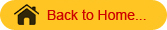INVERSE OF A MATRIX
Definition
Let A be any square matrix.  If there exists another square matrix B Such that AB = BA = I (I is a unit matrix) then B is called the inverse of the matrix A and is denoted by A-1.
The cofactor method is used to find the inverse of a matrix. Using matrices, the solutions of simultaneous equations are found.
Working Rule to find the inverse of the matrix
Step 1: Find the determinant of the matrix.
Step 2: If the value of the determinant is non zero proceed to find the inverse of the matrix.
Step 3: Find the cofactor of each element and form the cofactor matrix.
Step 4: The transpose of the cofactor matrix is the adjoint matrix.
Step 5:  The inverse of the matrix A-1 =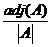Finding Matrix Inverse

Example
Find the inverse of the matrix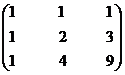Solution
Let A =Step 1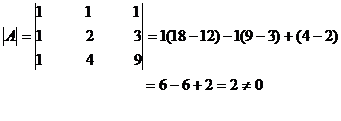Step 2
The value of the determinant is non zero
\A-1 exists.
Step 3
Let Aij denote the cofactor of aij in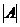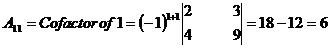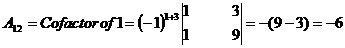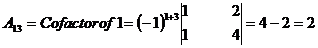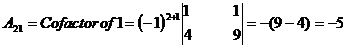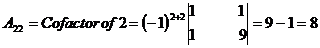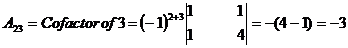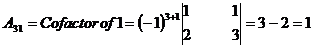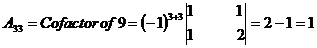Step 4
The matrix formed by cofactors of element of determinantis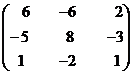\adj A =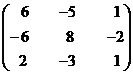Step 5=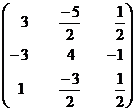SOLUTION OF LINEAR EQUATIONS
Let us consider a system of linear equations with three unknownsThe matrix form of the equation is AX=B where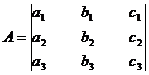is a 3x3 matrix
X =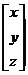and B =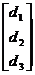Here AX = B
Pre multiplying both sides by A‑1.
(A-1 A)X= A-1B
We know that A-1 A= A A-1=I
\ I X= A-1B
since IX = X
Hence the solution is X =  A-1B.
Example
Solve the x + y + z = 1, 3x + 5y + 6z = 4, 9x + 26y + 36z =16 by matrix method.
Solution
The given equations are    x + y + z = 1,
3x + 5y + 6z = 4,
9x + 26y + 36z =16

Let A=, X=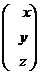, B=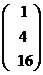The given system of equations can be put in the form of the matrix equation AX=B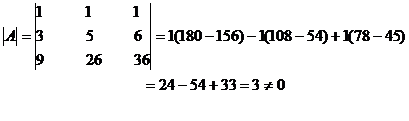The value of the determinant is non zero
\ A-1 exists.
Let Aij (i, j = 1,2,3) denote the cofactor of aij in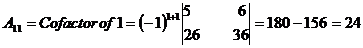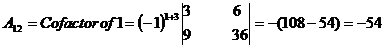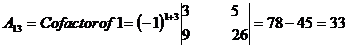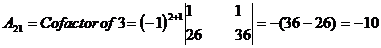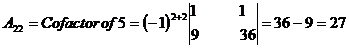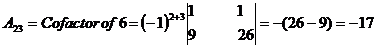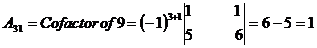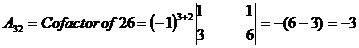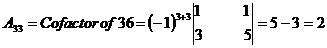The matrix formed by cofactors of element of determinantis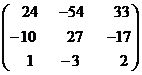\adj A =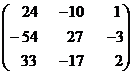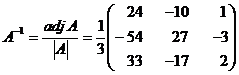We Know that      X=A-1B=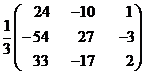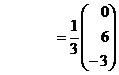=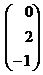x = 0, y = 2, z = -1.
SOLUTION BY DETERMINANT (CRAMER'S RULE)
Let the equations be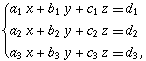…………………….  (1)
Consider the determinant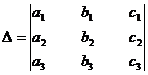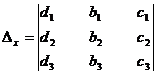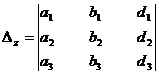When D ≠ 0, the unique solution is given by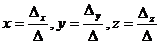Example
Solve the equations x + 2y + 5z =23, 3x + y + 4z = 26,                           6x + y + 7z = 47 by determinant method (Cramer’s Rule).
Solution
The equations are
x + 2y + 5z =23,
3x + y + 4z = 26,
6x + y + 7z = 47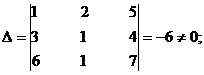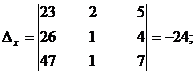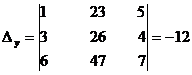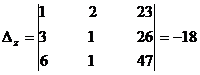By Cramer’s rule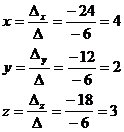Þ x = 4, y = 2, z = 3.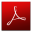Download this lecture as PDF here